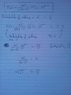# [Statistical Physics] No. of dice rolls so prob of getting a 6 is >90%

Flucky
This question is really wearing me out, any ideas?

## Homework Statement

How many times must I throw a fair dice in order to make the probability of getting at least one ‘6’ greater than 90%?
With this many throws, what is the probability of getting three ‘6’s?

It's the first part I'm struggling with.

## Homework Equations

The relevant question is the one on the top of the pic attached.

Where
P = probability of outcome interested in
N = number of throws

## The Attempt at a Solution

Attatched.

I'm using P(1) as the probability of getting 1 six, so n=1.
For the second part of the equation I would use P(3).

#### Attachments

•stats Q.jpg
16.5 KB · Views: 456

dauto
You are trying to calculate the probability of getting one 6. The question is what's the probability of getting AT LEAST one six. that includes two 6s, three 6s, etc. Try calculating the probability of getting NO 6s. That should be 10% since 90% of the time you're getting at least one 6.

•1 person
Homework Helper
Gold Member
You want to consider the probability of getting at least one six. That would include the probability of getting two sixes, or three sixes, ... or N sixes.

How does the probability of getting at least one six in N throws relate to the probability of getting no sixes in N throws?

•